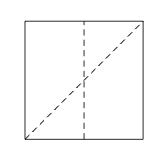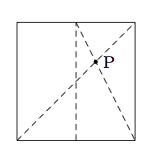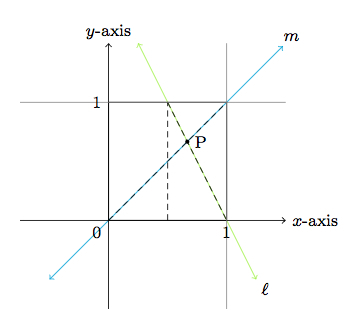# Folding a Square into Thirds

Alignments to Content Standards: 8.EE.B 8.EE.C.8

Suppose we take a square piece of paper and fold it in half vertically and diagonally, leaving the creases shown below:Next we make a fold that joins the top of the vertical crease to the bottom right corner, leaving the crease shown below. The point $P$ is the intersection of this new crease with the first diagonal fold.1. Place the lower left corner of the square at (0,0) on a coordinate grid with the upper right corner at (1,1) as pictured below:The lines $\ell$ and $m$ labelled in the picture contain the two diagonal folds. Find equations defining $\ell$ and $m$ and use these to calculate the coordinates of the point $P$.

2. Explain how to use part (a) in order to fold the square into thirds.

## IM Commentary

The purpose of this task is to find and solve a pair of linear equations which can be used to understand a common method of folding a square piece of origami paper into thirds. It is important for students to do the actual paper folding: if square shaped paper is not available then they will have an extra step to do, constructing a square from a rectangular piece of paper. The teacher may wish to emphasize the connection between reflections and folding a piece of paper: each fold makes a crease along a line segment and the ''fold'' is closely linked with the refection (of the plane containing the paper) along that line. An alternative geometric solution based on similar triangles is presented in www.illustrativemathematics.org/illustrations/1572. Much of this other task is aligned with 8th grade geometry standards (such as 8.G.5), but at this level the arguments involving similarity are supposed to be informal. The teacher may wish to discuss the geometric argument informally.

The two reflection lines associated to the folds of the square have relatively simple equations: students may be able to identify an equation defining $m$ right away, although finding an equation defining $\ell$ requires more careful manipulation. Students must both find, using the given graphs, the equations defining the two lines (8.EE.C) and then solve a simultaneous pair of linear equations (8.EE.C.8). The concrete setting and relatively simple coefficients should help facilitate work on this task, but it does have multiple parts and students need to be given ample time to complete it.

One practice standard closely related to this task is MP.2, Reason Abstractly and Quantitatively. Students have to translate back and forth between the physical paper folding and the mathematical representation of the paper in the coordinate plane. Another relevant standard of practice is MP.5, Use Appropriate Tools Strategically. This applies both to the fact that the task involves paper folding and to the fact that a computer could be helpful for drawing pictures and verifying the accuracy of the solution.

## Solution

1. For the line $m$ we can see that this is defined by the equation $y = x$: it has slope 1 since it passes through (0,0) and (1,1) and the $y$-intercept is 0 since it passes through (0,0). For the line $\ell$, we can calculate the slope using the information given. Since $\ell$ contains the points $\left(\frac{1}{2},1\right)$ and $(1,0)$ its slope is $$\frac{1-0}{\frac{1}{2} - 1} = \frac{1}{-\frac{1}{2}} = -2.$$ To find the $y$-intercept of this line, note that going from (1,0) to $\left(\frac{1}{2},1\right)$ we have moved left $\frac{1}{2}$ and up 1. If we move left $\frac{1}{2}$ and up 1 a second time we will be at the point $(0,2)$. This means that the $y$-intercept of $\ell$ is 2. Putting together what we know about the slope and $y$-intercept, an equation defining $\ell$ is $$y = -2x + 2.$$

To solve $y = x$ and $y = -2x + 2$ simultaneously we can first plug in $y = x$ to $y = -2x + 2$ giving $$x = -2x + 2.$$ Solving for $x$ gives $x = \frac{2}{3}$. Plugging this into either of our equations gives $y = \frac{2}{3}$. So the point $P$ in common to $\ell$ and $m$ is $\left(\frac{2}{3},\frac{2}{3}\right)$.

2. If we fold the left side of the square over to $P$ so that the left edge remains parallel to the right edge we will have the additional vertical crease shown in the figure below. This crease will be $\frac{1}{3}$ of the width of the square since it is halfway from the left edge to $P$ and, from part (a), the $x$-coordinate of $P$ is $\frac{2}{3}$:We could then fold the right hand side of the square over to the purple dotted line to get a vertical crease going through $P$, dividing the square into three equal vertical sections, each of width $\frac{1}{3}$.